• ## PCA降维

千次阅读 2017-08-24 09:48:45
PCA降维
PCA降维

MDS降维在完全保持距离的情况下，推导式严谨的，而当需要损失一定维度的时候，直接去掉特征值小的维度就用到了主成分分析的方法。PCA降维是高等代数里面的一个技巧，属于各个领域应用比较多的一种降维方法。但是西瓜书上的推导过于粗略，很多线性代数或者矩阵求导的部分都直接给出了结果或者跳步，所以我自己详细推导了一下。

PCA降维优化目标的两种推出方式

首先，我们推出PCA降维的思路是投影到一个低维度的子空间中，这一点和MDS降维的精确形式一致。那么，怎样的子空间是优秀的呢？有以下两种想法:
1)最近重构性，投影离原来向量尽量接近。
2)最大可分性，投影之间尽量分开。

其实还有一种想法是如果本来有两类，投影开来区分度要大，也就是说两类内部尽量接近，差得尽量远，就是广义瑞利熵刻画的，这就是LDA，但是这里没有两类，所以这种想法不考虑。

PCA降维导出目标函数的推理

西瓜书或者大部分教材对此推理的线性代数运算跳步很严重，很多并不显然的步骤被跳步了。比如以下几个技巧，把标量做转置，标量与向量换位，这些技巧如果不注意使用的话，很容易合并不了同类项无法进一步计算。
经过推理可以看出，两种思路推出的目标函数或者优化的形式是一致的。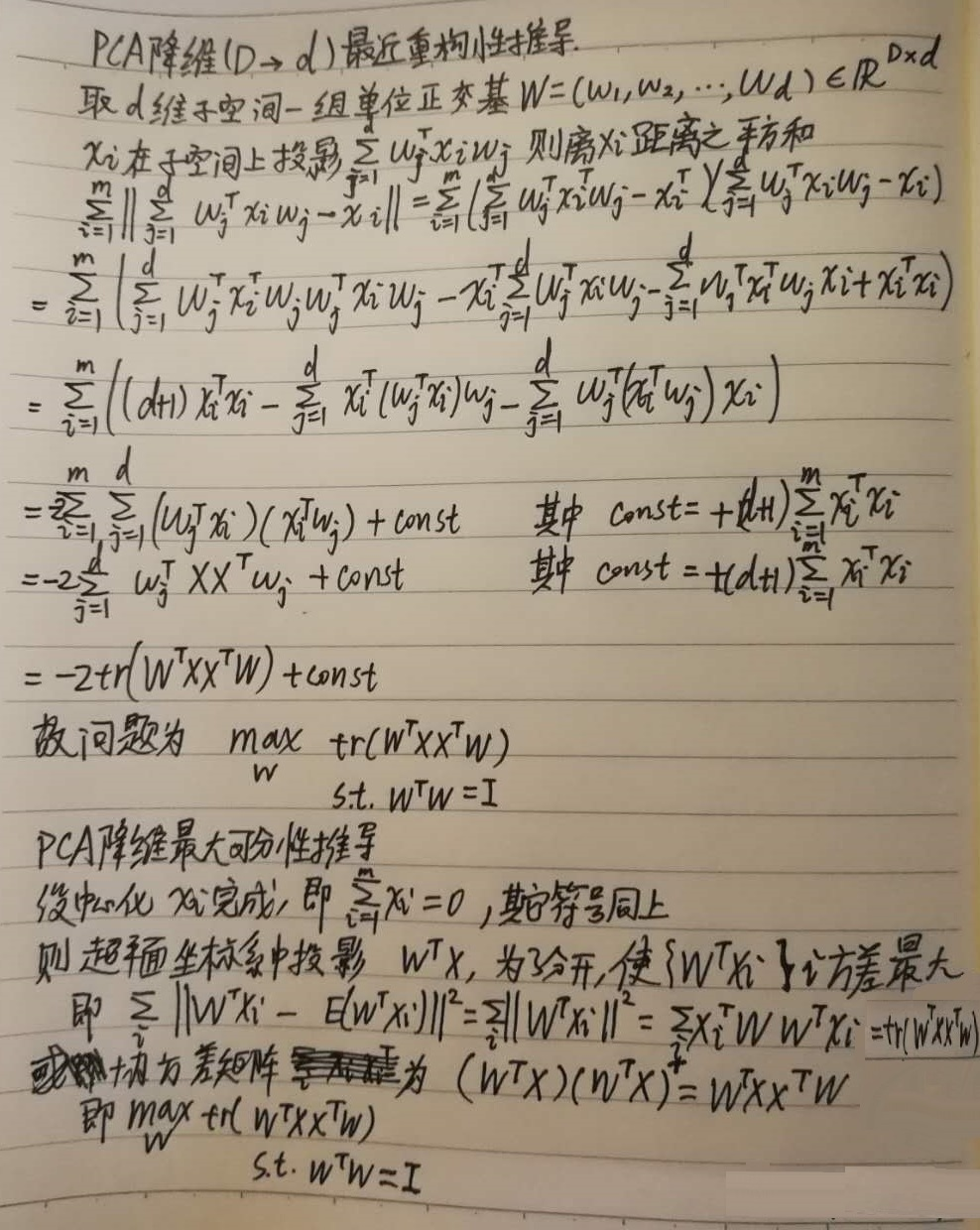优化函数的求解和中心化

目标函数的求解可以使用拉格朗日乘子法，矩阵求导的部分技巧还是很多的。如果不追求严格性的话，可以直接把矩阵当作标量运算，再通过加转置符号平衡维度。以下我给出一个严格的证明，展开一个维度来计算，避免了纯标量的下标繁琐的问题。
另外，拉格朗日乘子法仅仅给出了驻点，为什么在诸多驻点中选择特征值大的那几个，我也通过驻点代入优化函数的值为特征值之和给出了解释。
另外，PCA保留信号一定百分比的能量，为什么是特征值之和保留一定百分比即可，是因为驻点代入优化函数的值为特征值之和，而优化函数的几何意义方差，也就是信号的能量。所以PCA降维要保留一定百分比的能量，只需要取特征值的和超过一定百分比即可。
最后还有一个中心化的小技巧。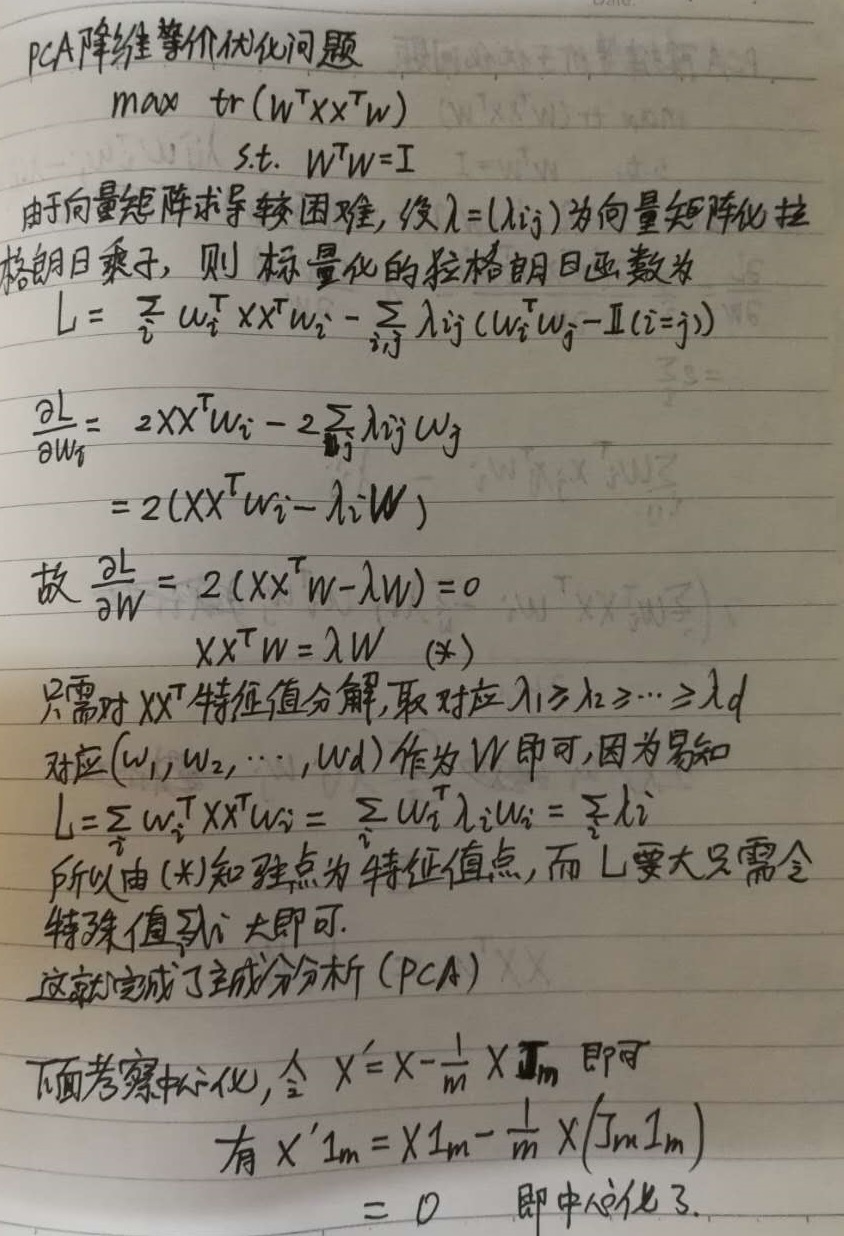展开全文• PCA降维 让你一看就懂的PCA降维工程 可运行
• PCA降维MATLAB程序,主要用于光谱的降维PCA降维MATLAB程序,主要用于光谱的降维pc
• PCA 简化数据 通俗理解: 找出一个最主要的特征 进行分析 例子: 考察一个人的智力情况 直接看数学成绩就行 例子: 观看电视 将显示器的百万像素转化为一个三维图像 重点: 降维技术 主成分分析(PCA) 对半导体...
PCA 简化数据

通俗理解: 找出一个最主要的特征 进行分析
例子: 考察一个人的智力情况 直接看数学成绩就行
例子: 观看电视 将显示器的百万像素转化为一个三维图像
重点:
降维技术
主成分分析(PCA)
对半导体数据进行降维处理

降维技术

1.数据集更容易使用
2.降低算法的计算开销
3.去除噪声
4.使得结果易懂 ---(有利于可视化)


几种不同的降维方法:
1. 主成分分析(Principal Component Analysis, PCA)
通俗理解: 找出一个最主要的特征 进行分析
例子: 考察一个人的智力情况 直接看数学成绩就行
原理简介:
在PCA中,数据从原来的坐标系转化到了新的坐标系, 新坐标系的选择是由数据
本身决定的.
第一个新坐标轴选择的是原始数据中方差最大的方向
第二个新坐标轴的选择是和第一个坐标轴正交且具有最大方差的方向
该过程已知重复  重复次数为原始数据中特征的数目
会发现: 大部分方差后包含在最前面的几个新坐标轴中 因此可以忽略余下的坐标轴 即对数据进行降维处理

2. 因子分析(Factor Analysis)
通俗理解: 将多个实测变量转化为少数几个综合指标   反映一种降维的思想: 通过降维将相关性高的变量聚在一起 从而减少需要分析的变量的数量 而减少问题分析的复杂性
例子: 考察一个人的整体情况 就组合3样成绩(隐变量), 看平均成绩就行
原理简介:
在因子分析中, 假设在观察数据的生成中有一些观察不到的隐变量
假设观察数据是这些隐变量和某些噪声的线性组合 那么隐变量的数据可能比观察数据的数目少
也就是说找到隐变量就可以实现数据的降维

3.独立成分分析(Independent Component Analysis, ICA)
通俗理解: ICA认为观测信号是若干个独立信号的线性组合 ICA要做的就是解混过程
例子: 去KTV唱歌 想辨别唱的是什么歌曲? ICA是观察发现原唱唱的一首歌(2个独立的声音 (原唱/主唱))
ICA假设数据是从N个数据源生成的, 这一点和因子分析类似
假设数据为多个数据源的混合观察结构 这些数据源之间在统计上是相互独立的
在PCA中只假设数据是不相关的
如果数据源的数目少于观察数据的数目 则可以实现降维过程


PCA 主成分分析
优点:降低数据的复杂性  识别最重要的多个特征
缺点:不一定需要, 且可能损失有用信息
适用数据类型: 数值型数据

PCA是如何降维的? 移动坐标轴
1.1 移动坐标轴
第一条坐标轴旋转到覆盖数据的最大方差位置 —–> 数据的最大方差给出了数据的最重要的信息
第二条坐标轴是覆盖数据次大差异性的坐标轴

找到坐标轴之后如果降维?
坐标轴的旋转并没有减少数据的维度
考虑到决策树 每次都是基于一个特征来做决策的  分类规则简单 但分隔平面难以保持质量
考虑到SVM  分类规则复杂 但是分隔平面优异
PCA降维可以同时过得SVM和决策树的优点


PCA降维的基本过程
主成分分析
第一个主成分就是数据差异性最大的方向提取出来
第二个主成分则是来自于数据差异性次大的方向 并且该方向与第一个主成分方向正交
通过数据集的协方差矩阵以及特征值分析 就可以求出这些主成分的值

一旦得到了协方差矩阵的特征向量 就可以保留最大的N个值 这些特征向量也给出了N个最重要特征的真实结构
可以通过将数据乘上这N个特征向量 而将他转化到新的空间


特征值分析是线性代数中的一个领域 可以通过数据的一般格式来揭示数据的’真实’结构
即: 常说的特征向量和特征值
Av=λv中,v是特征向量λ是特征值特征值都是简单的标量值因此该公式表示的是:如果特征向量v被某个矩阵A左乘那么它就等于某个标量λ乘以v$Av=\lambda v中,v是特征向量\lambda 是特征值特征值都是简单的标量值因此该公式表示的是:如果特征向量v被某个矩阵A左乘那么它就等于某个标量\lambda 乘以v$$Av = \lambda v 中, v是特征向量 \lambda是特征值 特征值都是简单的标量值 因此该公式表示的是: 如果特征向量v被某个矩阵A左乘 那么它就等于某个标量\lambda 乘以 v$

PCA 工作原理
1. 找出第一个主成分的方向 也就是数据方差最大的方向
2. 找出第二个主成分的方向 也就是数据方差次大的方向   并且该方向与第一个主成分方向 正交( 如果是二维空间就叫垂直)
3. 通过这种方式计算出所有的主成分方向
4. 通过数据集的协方差矩阵的特征值和特征向量   可以得到这些主成分的值
5. 一旦得到了协方差矩阵的特征值和特征向量 就可以保留最大的N个特征 这些特征向量也给出了N个最重要特征的真实结构
就可以通过将数据乘上N个特征向量 从而将它转化到新的空间上

为什么正交?
1. 正交是为了数据的有效性损失最小
2. 正交的一个原因是特征值的特征向量是正交的


特征值与特征向量在代数和几何层面的实际意义是什么?
Ax = cx    A为矩阵 x为特征向量 c为特征值
矩阵A乘以x表示: 对向量x进行一次转化(旋转或者拉伸)是一种线性变换, 而该转换的效果为常数乘以向量x(即只进行拉伸)
通常: 求特征值和特征向量即为求出该矩阵能使哪些向量(当然是特征向量)只发生拉伸, 使其拉伸的程度如果(特征值大小)

意义:
看清一个矩阵在哪些方面能产生最大的效果
并根据所产生的每个特征向量(一般研究特征值最大的那几个)进行分类讨论与研究

算法实现 PCA 主成分分析
在数据转化为前N个主成分的伪代码大致如下:
去除平均值
计算协方差矩阵
计算协方差矩阵的特征值和特征向量
将特征值从大到小排序
保留最上面的N个特征向量
将数据转化到上述N个特征向量构建的新空间中

代码实现:

import numpy as np

print(__doc__)

"""
算法实现 PCA 主成分分析
在数据转化为前N个主成分的伪代码大致如下:
去除平均值
计算协方差矩阵
计算协方差矩阵的特征值和特征向量
将特征值从大到小排序
保留最上面的N个特征向量
将数据转化到上述N个特征向量构建的新空间中

"""
# def loadDataSet(fileName, delim='\t'):
#     fr = open(fileName)
#     stringArr = [line.strip().split(delim) for line in fr.readlines()]
#     datArr = [map(float, line) for line in stringArr]
#     return mat(datArr)

def load_data(filename, delim='\t'):
fr = open(filename)
data_set = list()
string_set = [line.strip().split(delim) for line in fr.readlines()]
# print('string_set', string_set)
for line in string_set:
data_set.append(list(map(float, line)))
return np.mat(data_set)

def pca(data_set, top_features=999999999):
"""
principal component analysis 主成分降维
:param data_set: 数据集
:param top_features: 去N个特征向量
:return:
low_data_mat   降维后的数据集
recon_mat      新的数据集空间

"""
# step1 去除均值并且计算协方差矩阵
data_mean = np.mean(data_set, axis=0)
# print('data_mean', data_mean)
mean_removed = data_set - data_mean

cov_mat = np.cov(mean_removed, rowvar=0)
# print('cov mat', cov_mat)
# step2 计算协方差矩阵的特征值和特征向量
eig_vals, eig_vects = np.linalg.eig(np.mat(cov_mat))
print('eig vals', eig_vals)
print('eig_vects', eig_vects)
# step3 将特征值从大到小排序 保留最上面的N个特征向量
eig_vals_index = np.argsort(eig_vals)   # 从小到大
# print('eig_vals_index', eig_vals_index)
eig_vals_index = eig_vals_index[:-(top_features + 1): -1]   # 从大到小
print('eig_vals_index', eig_vals_index)
read_eig_vects = eig_vects[:, eig_vals_index]    # 保留最上面的N个特征向量
# step4 将数据转换到新空间
low_data_mat = mean_removed * read_eig_vects   # 1000x2 * 2x1 = 1000x1
recon_mat = (low_data_mat * read_eig_vects.T) + data_mean
# print('low_data_mat', low_data_mat)
# print('recon_mat', recon_mat)
return low_data_mat, recon_mat

def mat_fig(data_set, recon_mat):
import matplotlib.pyplot as plt
figure = plt.figure()
ax = figure.add_subplot(111)
ax.scatter(data_set[:, 0].flatten().A, data_set[:, 1].flatten().A, marker='^', s=90)
ax.scatter(recon_mat[:, 0].flatten().A, data_set[:, 1].flatten().A, marker='o', s=50, c='r')
# figure.show()
plt.show()

def replace_nan_with_mean():
data_set = load_data('secom.data', ' ')
num_feature = np.shape(data_set)
for i in range(num_feature):
mean_val = np.mean(data_set[np.nonzero(~np.isnan(data_set[:, i].A)), i])
data_set[np.nonzero(np.isnan(data_set[:, i].A)), i] = mean_val
return data_set

def main():
# filename = 'testSet.txt'
# data_set = load_data(filename)
# print('data_set', data_set)
# low_data_mat, recon_mat = pca(data_set, top_features=1)
# print('降维后的数据集', np.shape(low_data_mat))
# print('low_data_mat', low_data_mat)
# print('新的数据集空间 recon_mat', recon_mat)
# mat_fig(data_set, recon_mat)
data_set = replace_nan_with_mean()
print('data_set', data_set)

if __name__ == '__main__':
main()

展开全文• PCA降维算法讲义ppt slides，pca降维算法，课程资源，ppt
• ## 通俗理解PCA降维作用

万次阅读 多人点赞 2017-08-13 16:37:05
本文主要介绍一种降维方法，PCA（Principal Component Analysis，主成分分析）。降维致力于解决三类问题。 1. 降维可以缓解维度灾难问题； 2. 降维可以在压缩数据的同时让信息损失最小化； 3. 理解几百个维度的数据...
概述
本文主要介绍一种降维方法，PCA（Principal Component Analysis，主成分分析）。降维致力于解决三类问题：

降维可以缓解维度灾难问题；
降维可以在压缩数据的同时让信息损失最小化；
理解几百个维度的数据结构很困难，两三个维度的数据通过可视化更容易理解。

下面，将从简介、计算步骤、应用三方面进行理解PCA的降维作用。
PCA简介
在理解特征提取与处理时，涉及高维特征向量的问题往往容易陷入维度灾难。随着数据集维度的增加，算法学习需要的样本数量呈指数级增加。有些应用中，遇到这样的大数据是非常不利的，而且从大数据集中学习需要更多的内存和处理能力。另外，随着维度的增加，数据的稀疏性会越来越高。在高维向量空间中探索同样的数据集比在同样稀疏的数据集中探索更加困难。
主成分分析 也称为 卡尔胡宁-勒夫变换（Karhunen-Loeve Transform），是一种用于探索高维数据结构的技术。PCA通常用于高维数据集的探索与可视化；还可以用于数据压缩，数据预处理等。PCA可以把可能具有相关性的高维变量合成线性无关的低维变量，称为主成分（ principal components）。新的低维数据集会尽可能的保留原始数据的变量。
PCA将数据投射到一个低维子空间实现降维。例如，二维数据集降维就是把点投射成一条线，数据集的每个样本都可以用一个值表示，不需要两个值。三维数据集可以降成二维，就是把变量映射成一个平面。一般情况下，$n$维数据集可以通过映射降成$k$维子空间，其中$k{\le}n$。
假如你是一本养花工具宣传册的摄影师，你正在拍摄一个水壶。水壶是三维的，但是照片是二维的，为了更全面的把水壶展示给客户，你需要从不同角度拍几张图片。下图是你从四个方向拍的照片：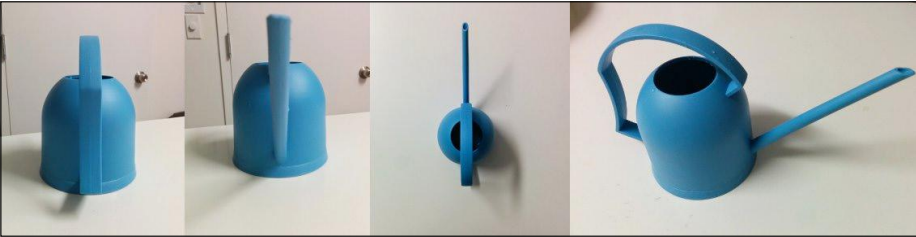第一张图里水壶的背面可以看到，但是看不到前面。第二张图是拍前面，可以看到壶嘴，这张图可以提供了第一张图缺失的信息，但是壶把看不到了。从第三张俯视图里无法看出壶的高度。第四张图是你真正想要的，水壶的高度，顶部，壶嘴和壶把都清晰可见。
PCA的设计理念与此类似，它可以将高维数据集映射到低维空间的同时，尽可能的保留更多变量。PCA旋转数据集与其主成分对齐，将最多的变量保留到第一主成分中。假设我们有下图所示的数据集：数据集看起来像一个从原点到右上角延伸的细长扁平的椭圆。要降低整个数据集的维度，我们必须把点映射成一条线。下图中的两条线都是数据集可以映射的，映射到哪条线样本变化最大？显然，样本映射到黑色虚线的变化比映射到红色点线的变化要大的多。实际上，这条黑色虚线就是第一主成分。第二主成分必须与第一主成分正交，也就是说第二主成分必须是在统计学上独立的，会出现在与第一主成分垂直的方向，如下图所示：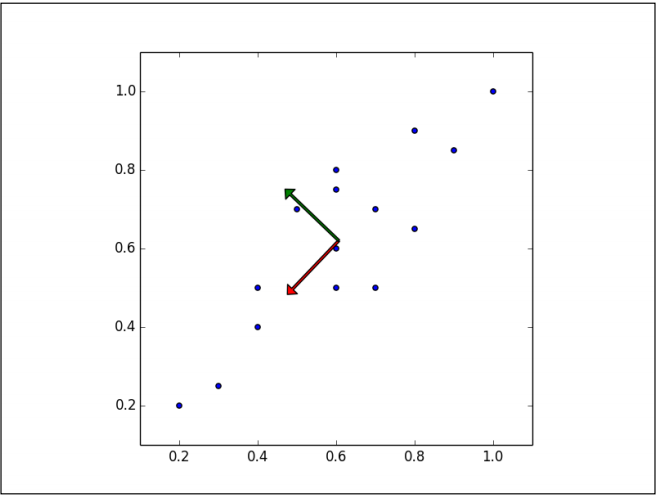后面的每个主成分也会尽量多的保留剩下的变量，唯一的要求就是每一个主成分需要和前面的主成分正交。
现在假设数据集是三维的，散点图看起来像是沿着一个轴旋转的圆盘。这些点可以通过旋转和变换使圆盘完全变成二维的。现在这些点看着像一个椭圆，第三维上基本没有变量，可以被忽略。
当数据集不同维度上的方差分布不均匀的时候，PCA最有用。（如果是一个球壳形数据集，PCA不能有效的发挥作用，因为各个方向上的方差都相等；没有丢失大量的信息维度一个都不能忽略）。
PCA的计算步骤
在介绍PCA的运行步骤之前，有一些术语需要说明一下。
方差，协方差和协方差矩阵
（对此概念不是很理解可以参考附录链接。）如何通俗易懂地解释「协方差」与「相关系数」的概念？中“GRAYLAMB”的回答。
方差（Variance） 是度量一组数据的分散程度。方差是各个样本与样本均值的差的平方和的均值：
$s^2 = \frac {\sum_{i=1}^n {{(X_i-\bar X)}^2}} {n-1}$
协方差（Covariance） 是度量两个变量的变动的同步程度，也就是度量两个变量线性相关性程度。如果两个变量的协方差为$0$，则统计学上认为二者线性无关。注意两个无关的变量并非完全独立，只是没有线性相关性而已。计算公式如下：
$cov(X,Y)=\frac {\sum_{i=1}^n {(X_i-\bar X)(Y_i-\bar Y)}} {n-1}$
如果协方差大于0表示一个变量增大是另一个变量也会增大，即正相关，协方差小于0表示一个变量增大是另一个变量会减小，即负相关。
协方差矩阵（Covariance matrix） 由数据集中两两变量的协方差组成。矩阵的第$(i,j)$个元素是数据集中第$i$和第$j$个元素的协方差。例如，三维数据的协方差矩阵如下所示：
$C= \begin{bmatrix} cov(x_1,x_1) & cov(x_1,x_2) & cov(x_1,x_3)\\ cov(x_2,x_1) & cov(x_2,x_2) & cov(x_2,x_3)\\ cov(x_3,x_1) & cov(x_3,x_2) & cov(x_3,x_3)\\ \end{bmatrix}$
让我们计算下表数据的协方差矩阵：
$\begin{array} {ccc} x_1 && x_2 && x_3\\ \hline 2 && 0 && -1.4 \\ 2.2 && 0.2 && -1.5 \\ 2.4 && 0.1 && -1 \\ 1.9 && 0 && -1.2 \\ \end{array}$
可以由python中的numpy包计算均值和协方差：
import numpy as np
X = [[2, 0, -1.4],
[2.2, 0.2, -1.5],
[2.4, 0.1, -1],
[1.9, 0, -1.2]]
print(np.mean(X,axis=0))
print(np.cov(np.array(X).T))

得到三个变量的样本均值分别是2.125，0.075和-1.275；协方差矩阵为：
$\begin{bmatrix} 0.04916667 & 0.01416667 & 0.01916667 \\ 0.01416667 & 0.00916667 & -0.00583333 \\ 0.01916667 & -0.00583333 & 0.04916667 \\ \end{bmatrix}$
特征向量和特征值
（可以直观的理解：“特征向量是坐标轴，特征值是坐标”）
向量是具有 大小（magnitude） 和 方向（direction） 的几何概念。
特征向量（eigenvector） 是由满足如下公式的矩阵得到的一个非零向量：$A \vec \nu = \lambda \vec \nu$其中，$\vec \nu$是特征向量，$A$是方阵，$\lambda$是特征值。经过$A$变换之后，特征向量的方向保持不变，只是其大小发生了特征值倍数的变化。也就是说，一个特征向量左乘一个矩阵之后等于等比例放缩（scaling）特征向量。德语单词eigen的意思是：“属于…或…专有（ belonging to or peculiar to）”；矩阵的特征向量是属于并描述数据集结构的向量。
特征向量和特征值只能由方阵得出，且并非所有方阵都有特征向量和特征值。如果一个矩阵有特征向量和特征值，那么它的每个维度都有一对特征向量和特征值。矩阵的主成分是由其协方差矩阵的特征向量，按照对应的特征值大小排序得到的。最大的特征值就是第一主成分，第二大的特征值就是第二主成分，以此类推。
让我们来计算下面矩阵的特征向量和特征值：
$A=\begin{bmatrix}1 & -2 \\2 & -3 \\\end{bmatrix}$
根据前面的公式$A$乘以特征向量，必然等于特征值乘以特征向量。我们建立特征方程求解：
$(A- \lambda I) \vec \nu=0$
$|A-\lambda * I| = \begin{vmatrix}\begin{bmatrix}1 & -2 \\2 & -3 \\\end{bmatrix}-\begin{bmatrix}\lambda & 0 \\0 & \lambda \\\end{bmatrix}\end{vmatrix}= 0$
从特征方程可以看出，矩阵与单位矩阵和特征值乘积的矩阵行列式为0，即：$\begin{vmatrix}\begin{bmatrix}1-\lambda & -2 \\2 & -3-\lambda\\\end{bmatrix}\end{vmatrix}=(\lambda+1)(\lambda+1)= 0$
矩阵的两个特征值都等于-1。现在再用特征值来解特征向量。
把$\lambda=-1$代入：
$(A- \lambda ) \vec \nu=0$
得：
\begin{aligned} \begin{pmatrix} \begin{bmatrix}1 & -2 \\ 2 & -3 \end{bmatrix} - \begin{bmatrix} \lambda & 0 \\ 0 & \lambda \end{bmatrix} \end{pmatrix}\vec\nu & = \begin{bmatrix} 1-\lambda & -2 \\ 2 & -3-\lambda \end{bmatrix}\vec\nu \\ & = \begin{bmatrix}1-\lambda & -2 \\2 & -3-\lambda \end{bmatrix} \begin{bmatrix}\nu_{1,1} \\ \nu_{1,2} \end{bmatrix} \\ & = \begin{bmatrix}1-(-1) & -2 \\ 2 & -3-(-1) \end{bmatrix} \begin{bmatrix}\nu_{1,1} \\ \nu_{1,2} \end{bmatrix} \\ & = \begin{bmatrix} 2 & -2 \\ 2 & -2 \end{bmatrix} \begin{bmatrix} \nu_{1,1} \\ \nu_{1,2} \end{bmatrix} \\ & = 0 \end{aligned}
所以有：
$\begin{cases} 2\nu_{1,1} - (2\nu_{1,2})=0 \\ 2\nu_{1,1}-(2\nu_{1,2})=0 \end{cases}$
任何满足方程$（A \vec \nu = \lambda \vec \nu）$的非零向量（取$\vec\nu=\begin{bmatrix}1\\\ 1\end{bmatrix}$）都可以作为特征向量：
$\begin{bmatrix} 1 & -2 \\ 2 & -3 \end{bmatrix} \begin{bmatrix}1\\1\end{bmatrix}= \begin{bmatrix}-1\\-1\end{bmatrix}= -1×\begin{bmatrix}1\\ 1\end{bmatrix}$
PCA需要单位特征向量，也就是$L_2$范数$\begin{Vmatrix}x \\\end{Vmatrix}=\sqrt {x_1^2 + x_2^2 + \dots + x_n^2}$等于1的特征向量。
于是有：$\begin{Vmatrix}\begin{bmatrix}1 \\1 \\\end{bmatrix}\end{Vmatrix}=\sqrt {1^2+1^2}=\sqrt 2$
对应的单位特征向量是：$\begin{bmatrix} 1 \\ 1 \\ \end{bmatrix} / {\sqrt 2}=\begin{bmatrix}0.70710678 \\0.70710678 \\\end{bmatrix}$
这里可以通过 numpy 检验手算的特征向量是否正确。eig 函数返回特征值和特征向量的元组：
import numpy as np
w, v = np.linalg.eig(np.array([[1, -2], [2, -3]]))
print('特征值：{}\n特征向量：{}'.format(w,v))

输出（这里特征值不同为1，是由于python编译器对浮点数据精度要求所致）：
特征值: [-0.99999998 -1.00000002]
特征向量: 	[[ 0.70710678  0.70710678]
[ 0.70710678  0.70710678]]

用PCA降维
让我们用PCA方法把下表二维数据降成一维：
$\begin{array} {cc} x_1 && x_2 \\ \hline 0.9 && 1 \\ 2.4 && 2.6\\ 1.2 && 1.7\\ 0.5 && 0.7\\ 0.3 && 0.7\\ 1.8 && 1.4\\ 0.5 && 0.6\\ 0.3 && 0.6\\ 2.5 && 2.6\\ 1.3 && 1.1\\ \end{array}$
PCA第一步是用样本数据减去样本均值：
$\begin{array}{cc} x_1 && x_2 \\ \hline 0.9-1.17=-0.27 && 1-1.3=-0.3 \\ 2.4-1.17=1.23 && 2.6-1.3=1.3 \\ 1.2-1.17=0.03 && 1.7-1.3=0.4 \\ 0.5-1.17=-0.67 && 0.7-1.3=-0.6 \\ 0.3-1.17=-0.87 && 0.7-1.3=-0.6 \\ 1.8-1.17=0.63 && 1.4-1.3=0.1 \\ 0.5-1.17=-0.67 && 0.6-1.3=-0.7 \\ 0.3-1.17=-0.87 && 0.6-1.3=-0.7 \\ 2.5-1.17=1.33 && 2.6-1.3=1.3 \\ 1.3-1.17=0.13 && 1.1-1.3=-0.2 \\ \end{array}$
然后，我们计算数据的主成分。前面介绍过，矩阵的主成分是其协方差矩阵的特征向量按照对应的特征值大小排序得到的。主成分可以通过两种方法计算：第一种方法是计算数据协方差矩阵。因为协方差矩阵是方阵，所以我们可以用前面的方法计算特征值和特征向量。第二种方法是用数据矩阵的奇异值分解（singular value decomposition）来找协方差矩阵的特征向量和特征值的平方根。我们先介绍第一种方法，然后介绍scikit-learn的PCA实现，也就是第二种方法。上述数据集的解释变量协方差矩阵如下：
$C=\begin{bmatrix} 0.6867777778 & 0.6066666667 \\0.6066666667 & 0.5977777778\\\end{bmatrix}$
用前面介绍过的方法，特征值是1.25057433和0.03398123，单位特征向量是：
$\begin{bmatrix}0.73251454 & 0.68075138 \\0.68075138 & 0.73251454 \\\end{bmatrix}$
下面我们把数据映射到主成分上。第一主成分是最大特征值对应的特征向量，因此我们要建一个转换矩阵，它的每一列都是主成分的特征向量。如果我们要把5维数据降成3维，那么我们就要用一个3维矩阵做转换矩阵。在本例中，我们将把我们的二维数据映射成一维，因此我们只需要用特征向量中的第一主成分作为转换矩阵。最后，我们用数据矩阵右乘转换矩阵。下面就是第一主成分映射的结果：
$\begin{bmatrix}-0.27 & -0.3 \\1.23 & 1.3 \\0.03 & 0.4 \\-0.67 & 0.6 \\-0.87 & 0.6 \\0.63 & 0.1 \\-0.67 & -0.7 \\-0.87 & -0.7 \\1.33 & 1.3 \\0.13 & -0.2\\\end{bmatrix} \begin{bmatrix}0.73251454\\0.68075138\\\end{bmatrix}= \begin{bmatrix}-0.40200434 \\ 1.78596968 \\ 0.29427599 \\-0.08233391 \\-0.22883682 \\ 0.5295593 \\-0.96731071 \\-1.11381362 \\ 1.85922113 \\-0.04092339 \\\end{bmatrix}$
通过numpy包中的矩阵调用实现过程如下：
import numpy as np
x = np.mat([[ 0.9, 2.4, 1.2, 0.5, 0.3, 1.8, 0.5, 0.3, 2.5, 1.3],
[ 1, 2.6, 1.7, 0.7, 0.7, 1.4, 0.6, 0.6, 2.6, 1.1]])
x = x.T
T = x - x.mean(axis=0)
C = np.cov(x.T)
w,v = np.linalg.eig(C)
v_ = np.mat(v[:,0])		#每个特征值对应的是特征矩阵的每个列向量
v_ = v_.T		#默认以行向量保存，转换成公式中的列向量形式
y = T * v_
print(y)

其实到这可以结束了…
= = = = = = = = = = = = = = = =无耻分割线= = = = = = = = = = = = = = = = = =
PCA的运用
高维数据可视化
二维或三维数据更容易通过可视化发现模式。一个高维数据集是无法用图形表示的，但是我们可以通过降维方法把它降成二维或三维数据来可视化。
Fisher1936年收集了三种鸢尾花分别50个样本数据（Iris Data）：Setosa、Virginica、Versicolour。解释变量是花瓣（petals）和萼片（sepals）长度和宽度的测量值，响应变量是花的种类。鸢尾花数据集经常用于分类模型测试，scikit-learn中也有。让我们把iris数据集降成方便可视化的二维数据：
%matplotlib inline
import matplotlib.pyplot as plt
from sklearn.decomposition import PCA
from sklearn.datasets import load_iris

首先，我们导入鸢尾花数据集和PCA估计器。PCA类把主成分的数量作为超参数，和其他估计器一样，PCA也用fit_transform()返回降维的数据矩阵：
data = load_iris()
y = data.target
X = data.data
pca = PCA(n_components=2)
reduced_X = pca.fit_transform(X)

最后，我们把图形画出来：
red_x, red_y = [], []
blue_x, blue_y = [], []
green_x, green_y = [], []
for i in range(len(reduced_X)):
if y[i] == 0:
red_x.append(reduced_X[i])
red_y.append(reduced_X[i])
elif y[i] == 1:
blue_x.append(reduced_X[i])
blue_y.append(reduced_X[i])
else:
green_x.append(reduced_X[i])
green_y.append(reduced_X[i])
plt.scatter(red_x, red_y, c='r', marker='x')
plt.scatter(blue_x, blue_y, c='b', marker='D')
plt.scatter(green_x, green_y, c='g', marker='.')
plt.show()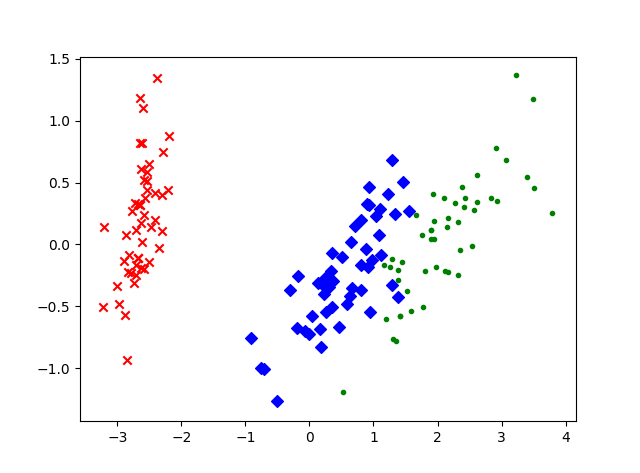降维的数据如上图所示。每个数据集中三个类都用不同的符号标记。从这个二维数据图中可以明显看出，有一个类与其他两个重叠的类完全分离。这个结果可以帮助我们选择分类模型。
脸部识别
现在让我们用PCA来解决一个脸部识别问题。脸部识别是一个监督分类任务，用于从照片中认出某个人。本例中，我们用剑桥大学AT&T实验室的 Faces数据集，这个数据集包含40个人每个人10张照片。这些照片是在不同的光照条件下拍摄的，每张照片的表情也不同。照片都是黑白的，尺寸为92 x 112像素。虽然这些图片都不大，但是每张图片的按像素强度排列的特征向量也有（92 x 112=）10304维。这些高维数据的训练可能需要很多样本才能避免拟合过度。而我们样本量并不大，所有我们用PCA计算一些主成分来表示这些照片。
我们可以把照片的像素强度矩阵转换成向量，然后用所有的训练照片的向量建一个矩阵。每个照片都是数据集主成分的线性组合。在脸部识别理论中，这些主成分称为特征脸（eigenfaces）。特征脸可以看成是脸部的标准化组成部分。数据集中的每张脸都可以通过一些标准脸的组合生成出来，或者说是最重要的特征脸线性组合的近似值。
from os import walk, path
import numpy as np
import mahotas as mh
from sklearn.cross_validation import train_test_split
from sklearn.cross_validation import cross_val_score
from sklearn.preprocessing import scale
from sklearn.decomposition import PCA
from sklearn.linear_model import LogisticRegression
from sklearn.metrics import classification_report
X = []
y = []

下面我们把照片导入Numpy数组，然后把它们的像素矩阵转换成向量：
for dir_path, dir_names, file_names in walk('C:/Users/HLB/Desktop/first blog/att_faces/'):
#walk() 函数内存放的是数据的绝对路径，同时注意斜杠的方向。
for fn in file_names:
if fn[-3:] == 'pgm':
image_filename = path.join(dir_path, fn)
X.append(scale(mh.imread(image_filename, as_grey=True).reshape(10304).astype('float32')))
y.append(dir_path)
X = np.array(X)

然后，我们用交叉检验建立训练集和测试集，在训练集上用PCA：
X_train, X_test, y_train, y_test = train_test_split(X, y)
pca = PCA(n_components=150)

我们把所有样本降到150维，然后训练一个逻辑回归分类器。数据集包括40个类；scikit-learn底层会自动用one versus all策略创建二元分类器：
X_train_reduced = pca.fit_transform(X_train)
X_test_reduced = pca.transform(X_test)
print('训练集数据的原始维度是：{}'.format(X_train.shape))
print('PCA降维后训练集数据是：{}'.format(X_train_reduced.shape))
classifier = LogisticRegression()
accuracies = cross_val_score(classifier, X_train_reduced, y_train)

训练集数据的原始维度是：(300, 10304)
PCA降维后训练集数据是：(300, 150)
最后，我们用交叉验证和测试集评估分类器的性能。分类器的平均综合评价指标（F1 score）是0.88，但是需要花费更多的时间训练，在更多训练实例的应用中可能会更慢。
print('交叉验证准确率是：{}\n{}'.format(np.mean(accuracies), accuracies))
classifier.fit(X_train_reduced, y_train)
predictions = classifier.predict(X_test_reduced)
print(classification_report(y_test, predictions))

最终的分析结果：
交叉验证准确率是：0.829757290513
[ 0.83035714  0.83333333  0.8255814 ]
precision    recall  f1-score   support
C:/Users/HLB/Desktop/first blog/att_faces/s1		1.00      1.00      1.00         1
C:/Users/HLB/Desktop/first blog/att_faces/s10       1.00      1.00      1.00         2
C:/Users/HLB/Desktop/first blog/att_faces/s11       1.00      1.00      1.00         2
C:/Users/HLB/Desktop/first blog/att_faces/s12       1.00      1.00      1.00         2
C:/Users/HLB/Desktop/first blog/att_faces/s13       1.00      1.00      1.00         4
C:/Users/HLB/Desktop/first blog/att_faces/s14       1.00      1.00      1.00         3
C:/Users/HLB/Desktop/first blog/att_faces/s15       1.00      1.00      1.00         2
C:/Users/HLB/Desktop/first blog/att_faces/s16       1.00      0.75      0.86         4
C:/Users/HLB/Desktop/first blog/att_faces/s17       1.00      1.00      1.00         4
C:/Users/HLB/Desktop/first blog/att_faces/s18       1.00      1.00      1.00         3
C:/Users/HLB/Desktop/first blog/att_faces/s19       1.00      1.00      1.00         3
C:/Users/HLB/Desktop/first blog/att_faces/s2       	1.00      1.00      1.00         2
C:/Users/HLB/Desktop/first blog/att_faces/s20       1.00      1.00      1.00         2
C:/Users/HLB/Desktop/first blog/att_faces/s21       1.00      1.00      1.00         2
C:/Users/HLB/Desktop/first blog/att_faces/s22       1.00      1.00      1.00         2
C:/Users/HLB/Desktop/first blog/att_faces/s23       1.00      1.00      1.00         4
C:/Users/HLB/Desktop/first blog/att_faces/s24       1.00      1.00      1.00         2
C:/Users/HLB/Desktop/first blog/att_faces/s25       1.00      1.00      1.00         4
C:/Users/HLB/Desktop/first blog/att_faces/s26       1.00      1.00      1.00         5
C:/Users/HLB/Desktop/first blog/att_faces/s27       0.50      1.00      0.67         1
C:/Users/HLB/Desktop/first blog/att_faces/s28       1.00      0.67      0.80         3
C:/Users/HLB/Desktop/first blog/att_faces/s29       1.00      1.00      1.00         2
C:/Users/HLB/Desktop/first blog/att_faces/s3      	1.00      1.00      1.00         1
C:/Users/HLB/Desktop/first blog/att_faces/s30       1.00      1.00      1.00         3
C:/Users/HLB/Desktop/first blog/att_faces/s31       1.00      1.00      1.00         3
C:/Users/HLB/Desktop/first blog/att_faces/s32       1.00      1.00      1.00         1
C:/Users/HLB/Desktop/first blog/att_faces/s33       1.00      1.00      1.00         1
C:/Users/HLB/Desktop/first blog/att_faces/s34       1.00      1.00      1.00         3
C:/Users/HLB/Desktop/first blog/att_faces/s35       1.00      1.00      1.00         2
C:/Users/HLB/Desktop/first blog/att_faces/s36       0.67      1.00      0.80         2
C:/Users/HLB/Desktop/first blog/att_faces/s37       0.50      1.00      0.67         1
C:/Users/HLB/Desktop/first blog/att_faces/s38       1.00      1.00      1.00         5
C:/Users/HLB/Desktop/first blog/att_faces/s39       1.00      1.00      1.00         3
C:/Users/HLB/Desktop/first blog/att_faces/s4      	1.00      1.00      1.00         1
C:/Users/HLB/Desktop/first blog/att_faces/s40       1.00      1.00      1.00         1
C:/Users/HLB/Desktop/first blog/att_faces/s5       	1.00      0.83      0.91         6
C:/Users/HLB/Desktop/first blog/att_faces/s6       	1.00      1.00      1.00         3
C:/Users/HLB/Desktop/first blog/att_faces/s7       	1.00      1.00      1.00         2
C:/Users/HLB/Desktop/first blog/att_faces/s8       	1.00      1.00      1.00         2
C:/Users/HLB/Desktop/first blog/att_faces/s9       	1.00      1.00      1.00         1
avg / total       0.98      0.97      0.97       100

总结
本文主要介绍PCA降维问题。高维数据不能轻易可视化。估计器训练高维数据集时，也可能出现维度灾难。通过主成分分析法缓解这些问题，将可能解释变量具有相关性的高维数据集，通过将数据映射到一个低维子空间，降维成一个线性无关的低维数据集。最后拓展用PCA将四维的鸢尾花数据集降成二维数据进行可视化；并将PCA用在一个脸部识别系统。
扩展阅读

如何通俗易懂地解释「协方差」与「相关系数」的概念？
线性代数中，特征值与特征向量在代数和几何层面的实际意义是什么？
7-dimensionality-reduction-with-pca
机器学习/周志华著.–北京：清华大学出版署，2016（2017.2重印）ISBN 978-302-42328-7


展开全文python 数据分析 数据挖掘...